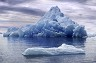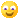# Urgent notice

1).if f(x) = x+1,.g(x) = x^2+1 fog (-2) =?

ans: 6.

2).if f(x-1) = x^2-4x+8 then f(x+1) = ?

ans : x^2+4

3).the value of 2logx+2logx^2+2logx^3 -----------------------nlogx^n =

ans: n(n+1)logx.

4).the recurring decimal 2.777 -----------is expressed as

ans: 25/9.

5).one root of x^2-3x+k = 0 is 2 then k is

ans: 2.

6).the value of k for which the roots of the equation 12x^2+kx+5 = 0 in the ratio 3:2.

ans: 5.10^1/2.Prakash (Chartered Accountant)     10 September 2011

Finally What do you want to Express Mam !!

Could't find anything to solve !!RAKESH (student)     10 September 2011

what is this?????????????????rohit (CA FINAL)     10 September 2011

isse kahte h attempt ka pressure "kya question hai...... lagta hi nahi ki question h....Ankit Hinduja (Student)     11 September 2011

for 1.f(x)=x+1,g(x)=x^2+1

so, f(g(x))=f(x^2+1)

=x^2+1+1

=x^2+2

now,f(-2)=(-2)^2+2(substituting x= -2 )

=4+2=6Ankit Hinduja (Student)     11 September 2011

for2.

2logx + 2log x^2 + 2logx^3+..........+nlogx^n

=2logx + 2.2logx + 2.3logx+.......+n.nlogx(by formula logx^n=nlogx)

=2logx(1+2+3+.....+n) ( taking out 2logx as common)

=2logx.n(n+1)/2  ( 1+2+3+....+n=n(n+1)/2)

=n(n+1)logx

if u r facing problem in maths u can refer maths books of 11th and 12th science group of any of ur science group friends .it will surely help..view more »